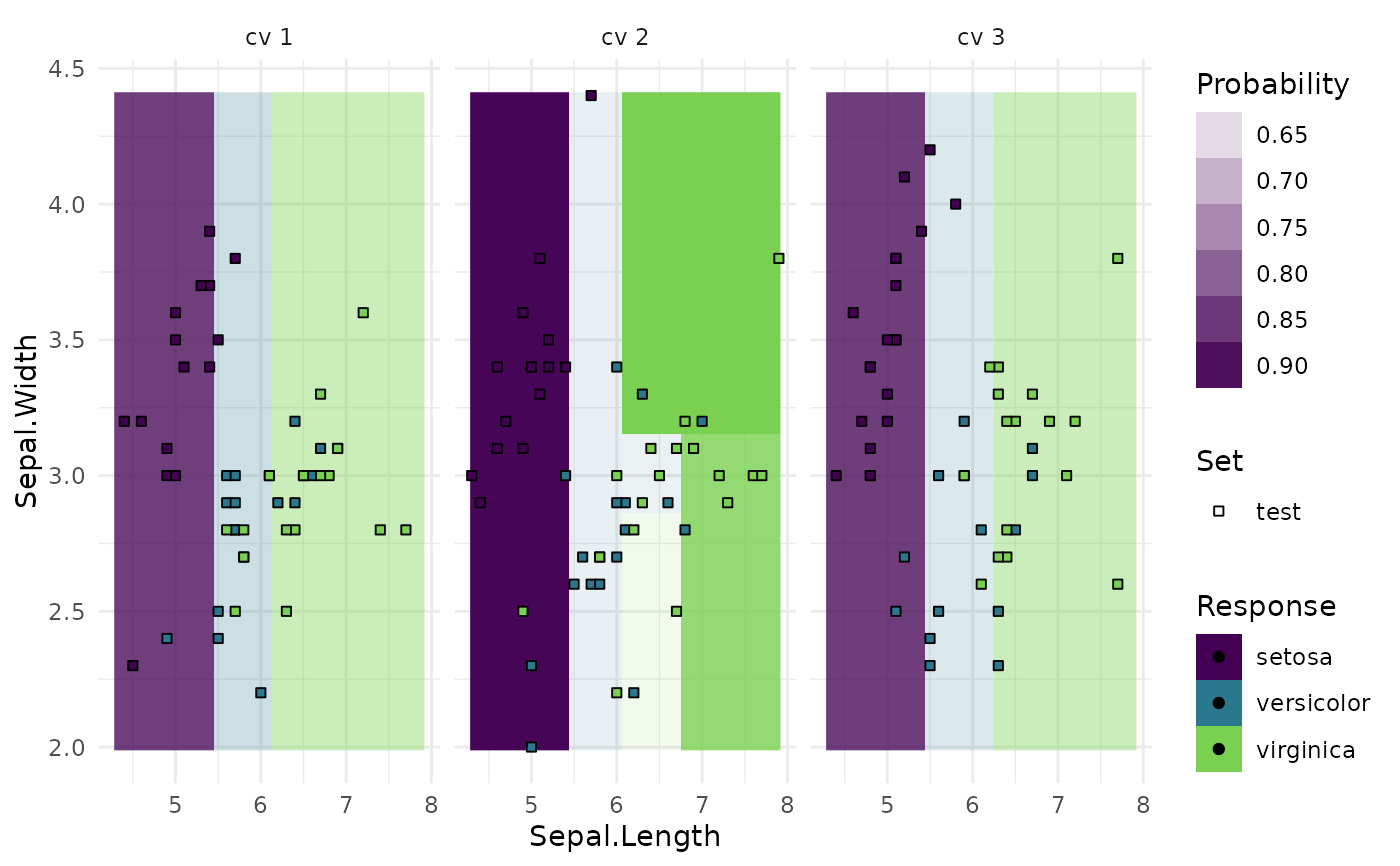Generates plots for mlr3::ResampleResult, depending on argument type:

• "boxplot" (default): Boxplot of performance measures.

• "histogram": Histogram of performance measures.

• "roc": ROC curve (1 - specificity on x, sensitivity on y). The predictions of the individual mlr3::Resamplings are merged prior to calculating the ROC curve (micro averaged). Requires package precrec.

• "prc": Precision recall curve. See "roc".

• "prediction": Plots the learner prediction for a grid of points. Needs models to be stored. Set store_models = TRUE for [mlr3::resample]. For classification, we support tasks with exactly two features and learners with predict_type= set to "response" or "prob". For regression, we support tasks with one or two features. For tasks with one feature we can print confidence bounds if the predict type of the learner was set to "se". For tasks with two features the predict type will be ignored.

# S3 method for ResampleResult
autoplot(object, type = "boxplot", measure = NULL, predict_sets = "test", ...)

## Arguments

object (character(1)): Type of the plot. See description. (character()) Only for type set to "prediction". Which points should be shown in the plot? Can be a subset of ("train", "test") or empty. (any): Additional arguments, passed down to the respective geom.

## Value

ggplot2::ggplot() object.

## Examples

library(mlr3)
library(mlr3viz)

learner = lrn("classif.rpart", predict_type = "prob")
resampling = rsmp("cv")

#> 1:         1 classif.ce   0.4285714
#> 2:         2 classif.ce   0.1904762
#> 3:         3 classif.ce   0.2380952
#> 4:         4 classif.ce   0.3333333
#> 5:         5 classif.ce   0.3333333
#> 6:         6 classif.ce   0.3333333
# Default: boxplot
autoplot(object)# Histogram
autoplot(object, type = "histogram", bins = 30)# ROC curve, averaged over resampling folds:
autoplot(object, type = "roc")# ROC curve of joint prediction object:
autoplot(object$prediction(), type = "roc")# Precision Recall Curve autoplot(object, type = "prc")# Prediction Plot task = tsk("iris")$select(c("Sepal.Length", "Sepal.Width"))
resampling = rsmp("cv", folds = 3)
object = resample(task, learner, resampling, store_models = TRUE)
autoplot(object, type = "prediction")